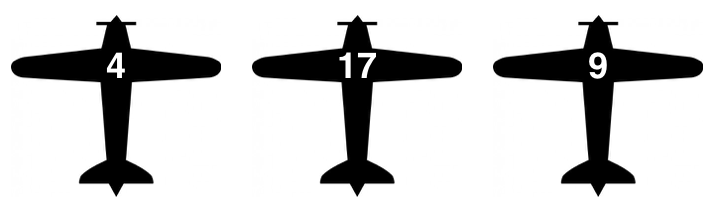# German Tank Problem

An airline has numbered their planes $1,2,\ldots,N,$ and you observe the following 3 planes, which are randomly sampled from the $N$ planes:What is the maximum likelihood estimate for $N?$ In other words, what value of $N$ would, according to conditional probability, make your observation most likely?

×